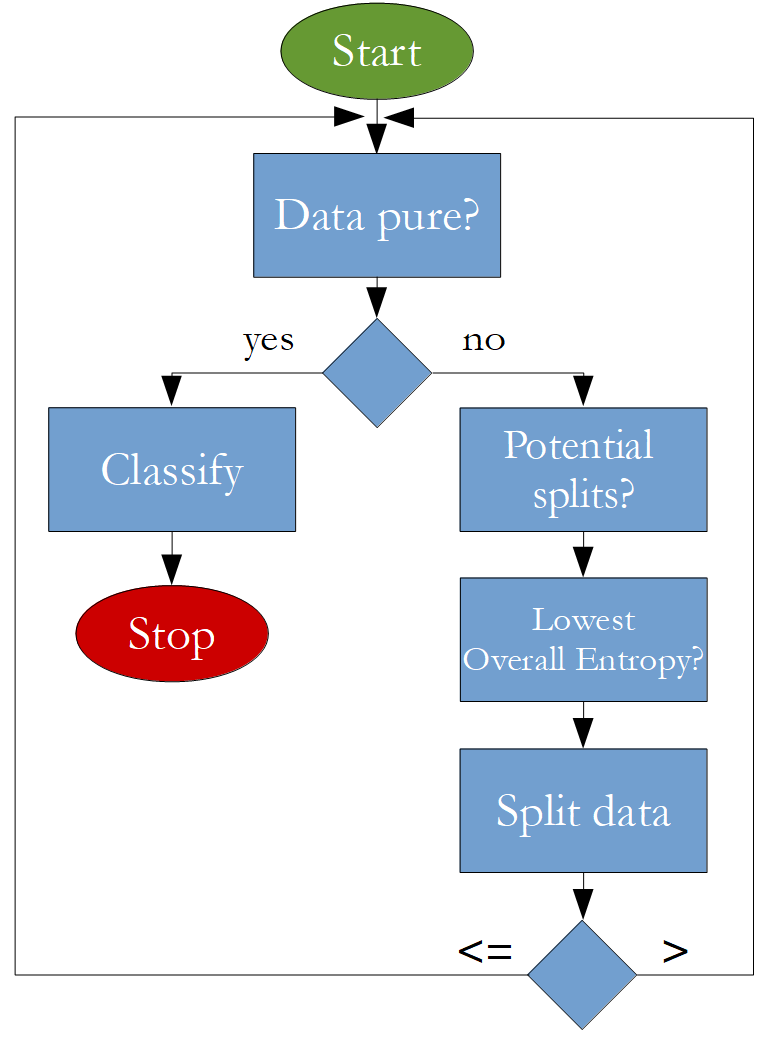The goal of this notebook is to code a decision tree classifier that can be used with the following API:

df = pd.read_csv("data.csv")

train_df, test_df = train_test_split(df, test_size=0.2)
tree = decision_tree_algorithm(train_df)
accuracy = calculate_accuracy(test_df, tree)


The algorithm that is going to be implemented looks like this:# Import Statements¶

In :
import numpy as np
import pandas as pd

import matplotlib.pyplot as plt
import seaborn as sns

import random
from pprint import pprint

In :
%matplotlib inline
sns.set_style("darkgrid")


#### Format of the data¶

• the last column of the data frame must contain the label and it must also be called "label"
• there should be no missing values in the data frame
In :
df = pd.read_csv("../data/Iris.csv")
df = df.drop("Id", axis=1)
df = df.rename(columns={"species": "label"})

In :
df.head()

Out:
sepal_length sepal_width petal_length petal_width label
0 5.1 3.5 1.4 0.2 Iris-setosa
1 4.9 3.0 1.4 0.2 Iris-setosa
2 4.7 3.2 1.3 0.2 Iris-setosa
3 4.6 3.1 1.5 0.2 Iris-setosa
4 5.0 3.6 1.4 0.2 Iris-setosa

# Train-Test-Split¶

In :
def train_test_split(df, test_size):

if isinstance(test_size, float):
test_size = round(test_size * len(df))

indices = df.index.tolist()
test_indices = random.sample(population=indices, k=test_size)

test_df = df.loc[test_indices]
train_df = df.drop(test_indices)

return train_df, test_df

In :
random.seed(0)
train_df, test_df = train_test_split(df, test_size=20)

In [ ]: